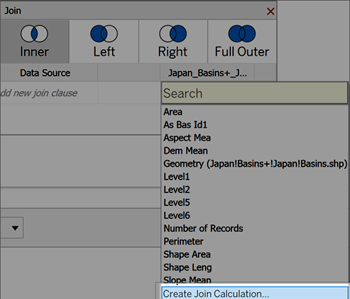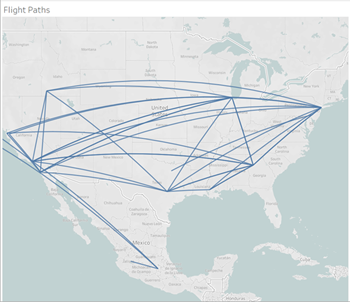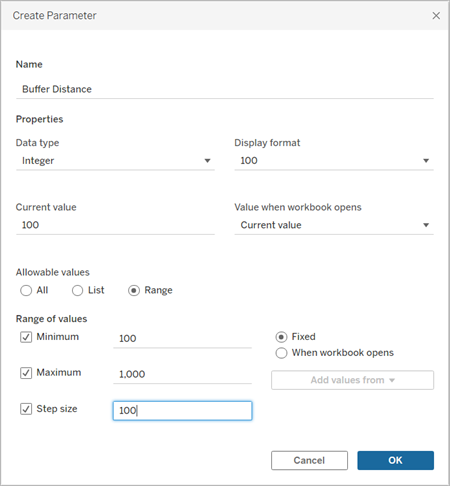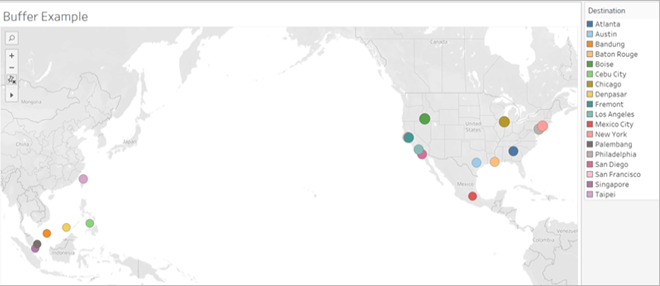# Spatial Functions

Spatial functions allow you to perform advanced spatial analysis and combine spatial files with data in other formats like text files or spreadsheets. For example, you might have a spatial file of city council districts, and a text file containing latitude and longitude coordinates of reported potholes. You can use a spatial calculation when creating your data source to join these files and analyze which district takes the longest to repair potholes.

You can also create a line that connects two data points for origin-destination maps. For example, you might have a spreadsheet of public transportation data that tells you where commuters began and ended their trips. You can use a spatial calculation to see what paths commuters are taking.

## Spatial functions available in Tableau

### AREA

 Syntax `AREA(Spatial Polygon, 'units')` Output Number Definition Returns the total surface area of a ``. Example `AREA([Geometry], 'feet')` Notes Supported unit names (must be in quotation marks in the calculation, such as `'miles'`): meters: meters, metres, mkilometers: kilometers, kilometres, kmmiles: miles, mifeet: feet, ft

### BUFFER

 Syntax `BUFFER(Spatial Point, distance, 'units')` Output Geometry Definition Returns a polygon shape centered over a ``, with a radius determined by the `` and `` values. Example `BUFFER([Spatial Point Geometry], 25, 'mi')``BUFFER(MAKEPOINT(47.59, -122.32), 3, 'km')` Notes Supported unit names (must be in quotation marks in the calculation, such as `'miles'`): meters: meters, metres, m kilometers: kilometers, kilometres, km miles: miles, mi feet: feet, ft

### DISTANCE

 Syntax `DISTANCE(SpatialPoint1, SpatialPoint2, 'units')` Output Number Definition Returns the distance measurement between two points in the specified ``. Example `DISTANCE([Origin Point],[Destination Point], 'km')` Notes Supported unit names (must be in quotation marks in the calculation, such as `'miles'`): meters: meters, metres, m kilometers: kilometers, kilometres, km miles: miles, mi feet: feet, ft Database limitations This function can only be created with a live connection but will continue to work if the data source is converted to an extract.

### INTERSECTS

 Syntax `INTERSECTS (geometry1, geometry2)` Output Boolean Definition Returns true or false indicating if two geometries overlap in space. Notes Supported combinations: point/polygon, line/polygon, and polygon/polygon.

### MAKELINE

 Syntax `MAKELINE(SpatialPoint1, SpatialPoint2)` Output Geometry (line) Definition Generates a line mark between two points Example `MAKELINE(MAKEPOINT(47.59, -122.32), MAKEPOINT(48.5, -123.1))` Notes Useful for building origin-destination maps.

### MAKEPOINT

 Syntax `MAKEPOINT(latitude, longitude, [SRID])` Output Geometry (point) Definition Converts data from `` and `` columns into spatial objects. If the optional `` argument is added, the inputs can be other projected geographic coordinates. Example `MAKEPOINT(48.5, -123.1)``MAKEPOINT([AirportLatitude], [AirportLongitude])``MAKEPOINT([Xcoord],[Ycoord], 3493)` Notes `MAKEPOINT` can't use the automatically generated latitude and longitude fields. The data source must contain the coordinates natively. `SRID` is a spatial reference identifier that uses ESPG reference system codes(Link opens in a new window) to specify coordinate systems. If `SRID` is not specified, WGS84 is assumed and parameters are treated as latitude/longitude in degrees. You can use `MAKEPOINT` to spatially-enable a data source so that it can be joined with a spatial file using a spatial join. For more information, see Join Spatial Files in Tableau .

### LENGTH

 Syntax `LENGTH(geometry, 'units')` Output Number Definition Returns the geodetic path length of the line string or strings in the `` using the given ``. Example `LENGTH([Spatial], 'metres')` Notes The result is `` if the geometry argument has no linestrings, though other elements are permitted.

### OUTLINE

 Syntax `OUTLINE(spatial polygon)` Output Geometry Definition Converts a polygon geometry into linestrings. Notes Useful for creating a separate layer for an outline that can be styled differently than the fill. Supports polygons within multipolygons.

### SHAPETYPE

 Syntax `SHAPETYPE(geometry)` Output String Definition Returns a string describing the structure of the spatial ``, such as Empty, Point, MultiPoint, LineString, MultiLinestring, Polygon, MultiPolygon, Mixed, and unsupported Example `SHAPETYPE(MAKEPOINT(48.5, -123.1)) = "Point"`

## Use a spatial calculation

### Create a spatial data source using MAKEPOINT

You can use MAKEPOINT to spatially-enable a data source so that it can be joined with a spatial file using a spatial join. To use MAKEPOINT, your data must contain latitude and longitude coordinates.

1. Open Tableau and connect to a spatial data source.
2. Under Connections, click Add to add a second, non-spatial data source.

The two data sources are added to the canvas.

Tip: To get the Join dialog box to appear, double-click (control click on Mac) a data source on the canvas.

3. Drag the non-spatial data source onto the Join dialog box.

4. Click the Join icon.
5. In the Join dialog box that appears, do the following:
• Select a join type.
• Under Data Source, select a spatial field from your spatial file to join by. Spatial fields have a globe icon next to them.
6. For the non-spatial data source, select Create Join Calculation as the join clause.The calculation should look something like this:

` MAKEPOINT(Latitude,Longitude)`

7. Seleck OK.
8. Select the Intersects join clause operator to create a data source for spatial analysis.

9. When finished, close the Join dialog box.

For more information on spatial joins, see Join Spatial Files in Tableau .

### Create a visualization using MAKELINE

In Tableau Desktop, download the Flight Path workbook from Tableau Public, available here(Link opens in a new window).

1. Navigate to a new worksheet.
2. Select Analysis > Create Calculated Field.
3. In the calculation that opens, do the following:
• Name the calculated field Flight Paths
• Enter the following formula

` MAKELINE(MAKEPOINT([Lat],[Lng]),MAKEPOINT([Dest Lat],[Dest Lng]))`

This formula takes latitude and longitude coordinates from your origin and destination cities and turns them into geographic points for spatial analysis. Those coordinates are used to build to two-point lines between origin and destination.

4. When finished, click OK.
5. The new calculated field appears under Dimensions in the Data pane. Just like your other fields, you can use it in one or more visualizations.

6. From the Data pane, double-click Flight Paths to add it to your visualization, which should automatically render as a map.
7.The calculation automatically produces curved geodesic lines when the lines span longer expanses of the globe.

### Visualize an area with BUFFER

In Tableau Desktop, download the Flight Path workbook from Tableau Public, available here(Link opens in a new window).

1. Navigate to a new worksheet.
2. Right-click the Data pane and select Create Parameter.
3. In the Parameter dialog that opens, set the options below:
• Name the parameter Buffer Distance
• Set the Data Type to Integer
• Set Allowable values to Range
• Set the Minimum range to 100, the Maximum range to 1000, and the step size to 100.4. When finished, click OK.
5. This parameter allows us to customize the radius of our buffer, ranging from 100 to 1000 miles. Right-click the parameter and select Show Parameter.

6. Select Analysis > Create Calculated Field.
7. In the calculation that opens, do the following:
• Name the calculated field Buffer
• Enter the following formula

` BUFFER(MAKEPOINT([Dest Lat],[Dest Lng]),[Buffer Distance],"miles")`

The BUFFER calculation takes point spatial data and converts it into shapes with a radius in miles determined by the Buffer Distance parameter.

Note: Because BUFFER can only be used with point spatial data, we're converting the latitude and longitude data into a point with Makepoint, as demonstrated in the previous example.

8. When finished, click OK.
9. The new calculated field appears in the Data pane. Just like your other fields, you can use it in one or more visualizations.

10. From the Data pane, double-click Buffer to add it to your visualization, which should automatically render as a map.
11. Drag Destination to the Color panel on the Marks card to complete the visualization.Note: If your view does not look like the image above, make sure that the Mark type is set to Map and not Circle. For more information on Marks type, see Change the Type of Mark in the View.